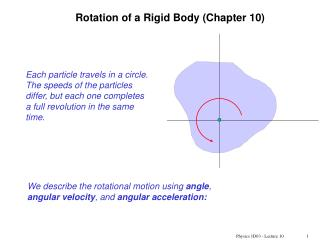DownloadDownload PresentationRotation of a Rigid Body (Chapter 10)

# Rotation of a Rigid Body (Chapter 10)

Download Presentation## Rotation of a Rigid Body (Chapter 10)

- - - - - - - - - - - - - - - - - - - - - - - - - - - E N D - - - - - - - - - - - - - - - - - - - - - - - - - - -
##### Presentation Transcript

1. Rotation of a Rigid Body (Chapter 10) Each particle travels in a circle. The speeds of the particles differ, but each one completes a full revolution in the same time. We describe the rotational motion using angle, angular velocity, and angular acceleration: Physics 1D03 - Lecture 10

2. Units: by convention, angles are measured in radians. arc length s r  r 2p rad = 360o Angular velocity has units of rad/s or s-1 Angular acceleration has units of rad/s2 or s-2 (The radian is a ratio of two lengths, and not really a unit. Some equations will require angles to be in radians.) Physics 1D03 - Lecture 10

3. (radians) (rad/s) (rad/s2 ) angle (“theta”): angular velocity (“omega”): angular acceleration (“alpha”): 0 reference axis Physics 1D03 - Lecture 10

4. v P  r s 0 circular path of point P Linear and angular quantities A particle P travels in a circle of radius r. The velocity is tangential to the circle and perpendicular to the radius. Distance:s = rq Tangential Velocity: Tangential Acceleration: Physics 1D03 - Lecture 10

5. Quiz The Earth rotates on its axis. How does its angular velocity w vary with location? a) w is larger at the equator, and smaller near the poles b) w is smaller at the equator, and larger near the poles c) w is the same at the equator and near the poles Physics 1D03 - Lecture 10

6. at P ar a In simpler notation: The tangential component at is equal to the rate of increase of speed. There is also a radial (centripetal) component, due to the change in direction ofv: These relations require angular quantities to be measured in radians (or rad/s, etc.). Physics 1D03 - Lecture 10

7. Quiz Several pennies are placed on a turntable. As the angular velocity of the turntable is slowly increased, which penny slides first? Physics 1D03 - Lecture 10

8. Quiz High-speed CD-ROM drives sometimes specify that they use a “constant linear velocity” method of recording. What does this mean for the rotation rate in revolutions per minute as the write head moves from the inner tracks to the outer tracks? Physics 1D03 - Lecture 10

9. Constant angular acceleration: All for constant a only ! These expressions are should remind you of relations for constant linear acceleration: qreplaces x, w replaces v, a replaces a. All for constant a only ! Physics 1D03 - Lecture 10

10. (Extra-derivation) Constant angular acceleration: In this special case, there are relations among q, w, and a that are analagous to the relations among x, v, and a for linear motion with constant linear acceleration. (constant a ; “initial” at t = 0) or or Physics 1D03 - Lecture 10

11. Example: A computer disc has a linear velocity of 1.3m/s. What is the angular velocity when at the innermost track where r=23mm. Physics 1D03 - Lecture 10

12. Example: A computer disc starts from rest and reaches a final rotation rate of 7200 rev/min after 10 seconds. Assuming constant angular acceleration, through how many revolutions does it turn? Physics 1D03 - Lecture 10

13. Summary • Rotational motion can be described by angle, angular velocity, and angular acceleration • For constant angular acceleration (a special case!), kinematical relations are similar to those for linear motion with constant linear acceleration Physics 1D03 - Lecture 10

14. Practice problems: Chapter 10Problems 1, 5, 11(5th ed) Problems 5, 7, 11 Physics 1D03 - Lecture 10